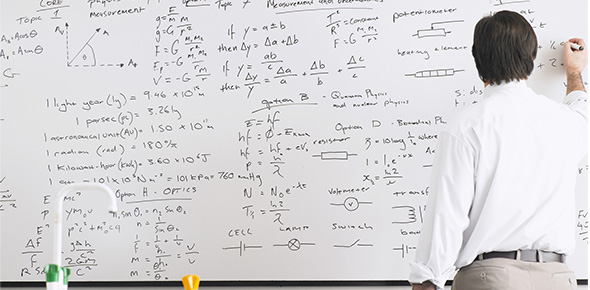# Section 4.2 - Solving Linear Equations Using Inverse Operations

10 Questions | Attempts: 314Settings• 1.
Which inverse operation should be applied first to solve 3x + 5 = 10?
• A.

• B.

Subtraction

• C.

Division

• D.

Multiplication

• 2.
Solve 5x + 3 =  23
• A.

X = 5.2

• B.

X = 20

• C.

X = 4

• D.

X = 6

• 3.
Solve   x/5 + 2 = 7
• A.

X = 25

• B.

X = 45

• C.

X = 1

• D.

X = 5

• 4.
Write an equation for the following problem, "Half of a number minus 6 is 20"
• A.

(x - 6) / 2 = 20

• B.

X - 6 = 20

• C.

2x - 6 = 20

• D.

X/2 - 6 = 20

• 5.
The equation C = 2.5p + 75 can be used to represent the cost, C to rent the rollar skating rink when p attend.  How much will it cost if 100 people attend.
• A.

\$250

• B.

\$325

• C.

\$75

• D.

\$175

• 6.
The equation P=2L + 2W can be used tp fomd the perimeter of a rectangle.  A rectangular shaped playground is enclosed by a fence that is 500m.  The length of the playground is 120m.  Use the equation to determine the width of the playground.
• A.

190m

• B.

260m

• C.

180m

• D.

130m

• 7.
The equation C = 10 + 0.25m represents the cost, C of a cell phone bill if m minutes are used.  Which equation represents a bill of \$25?
• A.

25 = 10 + 0.25m

• B.

C = 10 + 0.25(25)

• C.

C = 10 + 25

• D.

25 = 0.25m

• 8.
Solve x/4 + 6= 9
• A.

X = 4

• B.

X = 0.5

• C.

X = 60

• D.

X = 12

• 9.
Which inverse operation should be applied first to solve 5 - 3e = 15
• A.

• B.

Subtraction

• C.

Multiplication

• D.

Division

• 10.
Solve  (x - 3) / 5 = 10
• A.

X = 13

• B.

X = 65

• C.

X = 53

• D.

X = 47

## Related TopicsBack to top
×

Wait!
Here's an interesting quiz for you.